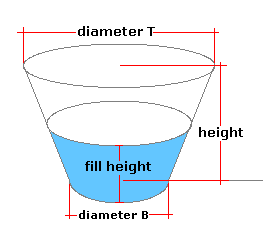Spike's Calculators

# Partial Volume in a Truncated Funnel Tank/Container - CentimetresCalculate the amount of liquid in a partially filled truncated funnel tank or container.

This calculator uses centimetres for measurements.

##### Approximate weight of Fuel or Water
```one litre of water = 1 kilogram
one litre of diesel fuel = 0.832 kilogram
one litre of gas = 0.75 kilogram
```

### Truncated Funnel

Top Diameter cm
Bottom Diameter cm
Funnel Height cm
Depth of Liquid cm
Liquid Weight-Kilograms per Litre kg/L

#### Results:

###### Tank Volume and Capacity
Cubic Centimetres cm³
Cubic Metres
Cubic Inches in³
Cubic Feet ft³
Capacity in Litres L
###### Amount of Liquid Inside Tank
In Litres L
In US Gallons gal US
In Imperial Gallons gal Imperial
In Cubic Metres
###### Weight of Liquid and Fill Amount
Weight of Liquid kg
To Fill this Tank, Add Litres

#### Calculator

1. enter the top diameter of the truncated funnel in centimetres
2. the bottom diameter in centimetres
3. the height of the funnel in centimetres
4. the depth of the liquid inside the tank in centimetres
5. the weight of the liquid in kilograms per litre

#### Results

1. the volume of the truncated funnel in cubic centimetres
2. the volume of the tank in cubic metres
3. the volume of the tank in cubic inches
4. the volume of the tank in cubic feet
5. the total amount of liquid the tank can hold in litres
6. the amount of liquid inside the tank in litres
7. the amount of liquid inside the tank in US gallons
8. the amount of liquid inside the tank in Imperial gallons
9. the amount of liquid inside the tank in cubic metres
10. the weight of the liquid inside the tank in kilograms
11. the amount of liquid it will take to top up this tank in litres

##### Formula
```V = d*π/3*p²+pa+a²
where V is the volume of the tank
d the fill height of the liquid
Π = 3.14159265
p diameter @ fill height
a half the tanks'  bottom diameter

```
##### Conversions
```one cubic centimetre (cm³) = 0.001 litres (L)
one cubic centimetre (cm³) = 0.000001 cubic metres (m³)
one cubic centimetre (cm³) = 0.0000353146667214886 cubic feet (ft³)
one cubic centimetre (cm³) = 0.0610237440947323 cubic inches (in³)
one cubic centimetre (cm³) = 0.000264172052358148 gallons (gal) US
one cubic centimetre (cm³) = 0.000219969248299088 gallons (gal) Imperial
```

### Funnel Tank/Container (Truncated)#### Filled